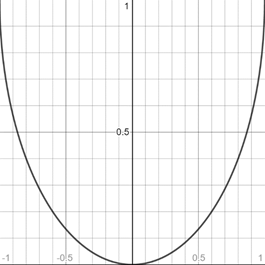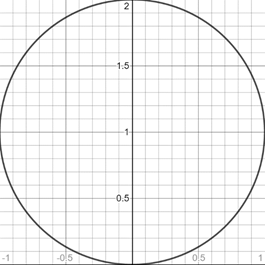# To graph : The circle ( y − 1 ) 2 + x 2 = 1 by solve for y .### Precalculus: Mathematics for Calcu...

6th Edition
Stewart + 5 others
Publisher: Cengage Learning
ISBN: 9780840068071### Precalculus: Mathematics for Calcu...

6th Edition
Stewart + 5 others
Publisher: Cengage Learning
ISBN: 9780840068071

#### Solutions

Chapter 1.9, Problem 28E
To determine

## To graph: The circle (y−1)2+x2=1 by solve for y .

Expert Solution

### Explanation of Solution

Given information:

The equation of circle (y1)2+x2=1 .

Graph:

The graph of circle (y1)2+x2=1 by solving for y .

Subtract x2 from the equation of circle,

(y1)2+x2x2=1x2(y1)2=1x2

Take under root on both side of the equation,

y1=±1x2

y1+1=1±1x2y=1±1x2

From the two equations graph of circle is described:

y=1+1x2,y=11x2

The graph of the equation y=1+1x2 represents upper half of the circle because y is always greater than or equal to 1.

1+1x211x201x2±1x

So, the values of x is less than or equal to ±1 .

Thus, the graph appropriate in the [1,1]×[0,2] viewing rectangle

The graph of the equation y=1+1x2 can be sketched using the table,

 x y 0 2 1 1 12 1+34

The graph of equation is provided below,The graph of the equation y=11x2 represents upper half of the circle because y is always less than or equal to 1.

11x211x201x20±1x

So, the values of x is greater than or equal to ±1 .

Thus, the graph appropriate in the [-1,1]×[0,1] viewing rectangle

The graph of the equation y=11x2 can be sketched using the table,

 x y 0 0 1 1 12 1−34

The graph of equation is provided below,The graph of both equations in same figure is provided below,

Thus, the graph appropriate in the [-1,1]×[0,2] viewing rectangleInterpretation:

The graph of an equation in a viewing screen is a viewing rectangle.

The x -values to range from a minimum value of xmin =a to a maximum value of xmax =b

The y -values to range from minimum value of ymin =c to a maximum value of ymax =d

Then, the display portion of the graph lies in the rectangle [a,b]×[c,d]={(x,y)|axb,cyd}

The graph of the equation y=1+1x2 represents upper half of the circle appropriate in the [1,1]×[0,1] viewing rectangle.

The graph of the equation y=11x2 represents upper half of the circle appropriate in the [1,1]×[0,2] viewing rectangle

The graph of the equation (y1)2+x2=1 represents circle in the [1,1]×[0,2] viewing rectangle.

### Have a homework question?

Subscribe to bartleby learn! Ask subject matter experts 30 homework questions each month. Plus, you’ll have access to millions of step-by-step textbook answers!